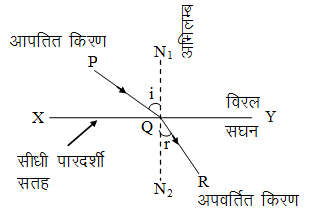# Physics

### Chapter : 4. Light Refraction

#### Refraction Of Light

Refraction Of Light
Definition : When light rays travelling in a medium are incident on a transparent surface of another medium they are bent as they travel in second medium.Fig. Refraction of light from a plane transparent denser surface.
SOME ASSOCIATED TERMS
Transparent surface : The plane surface which refracts light, is called transparent surface. In diagram, XY is the section of a plane transparent surface.
Point of incidence : The point on transparent surface, where the ray of light meets it, is called point of incidence. In diagram, Q is the point of incidence.
Normal : Perpendicular drawn on the transparent surface at the point of incidence, is called normal. In diagram, N1QN2 is the normal on surface XY.
Incident ray : The ray of light which strikes the transparent surface at the point of incidence, is called incident ray in diagram PQ is the incident ray.
Refracted ray : The ray of light which travels from the point of incidence into the other medium, is called refracted ray. In diagram, QR is the refracted ray.
Angle of incidence : The angle between the incident ray and the normal on the transparent surface at the point of incidence, is called the angle of incidence. It is represented by the symbol i. In diagram, angle PQN1 is the angle of incidence.
Angle of refraction : The angle between the refracted ray and the normal on the transparent surface at the point of incidence, is called angle of refraction. It is represented by symbol r. In diagram angle RQN2 is the angle of refraction.
Plane of incidence : The plane containing the normal and the incident ray, is called plane of incidence. For the diagram, plane of book page is the plane of incidence.
Plane of refraction : The plane containing the normal and the refracted ray, is called plane of refraction. For the diagram, plane of book page is the plane of refraction.